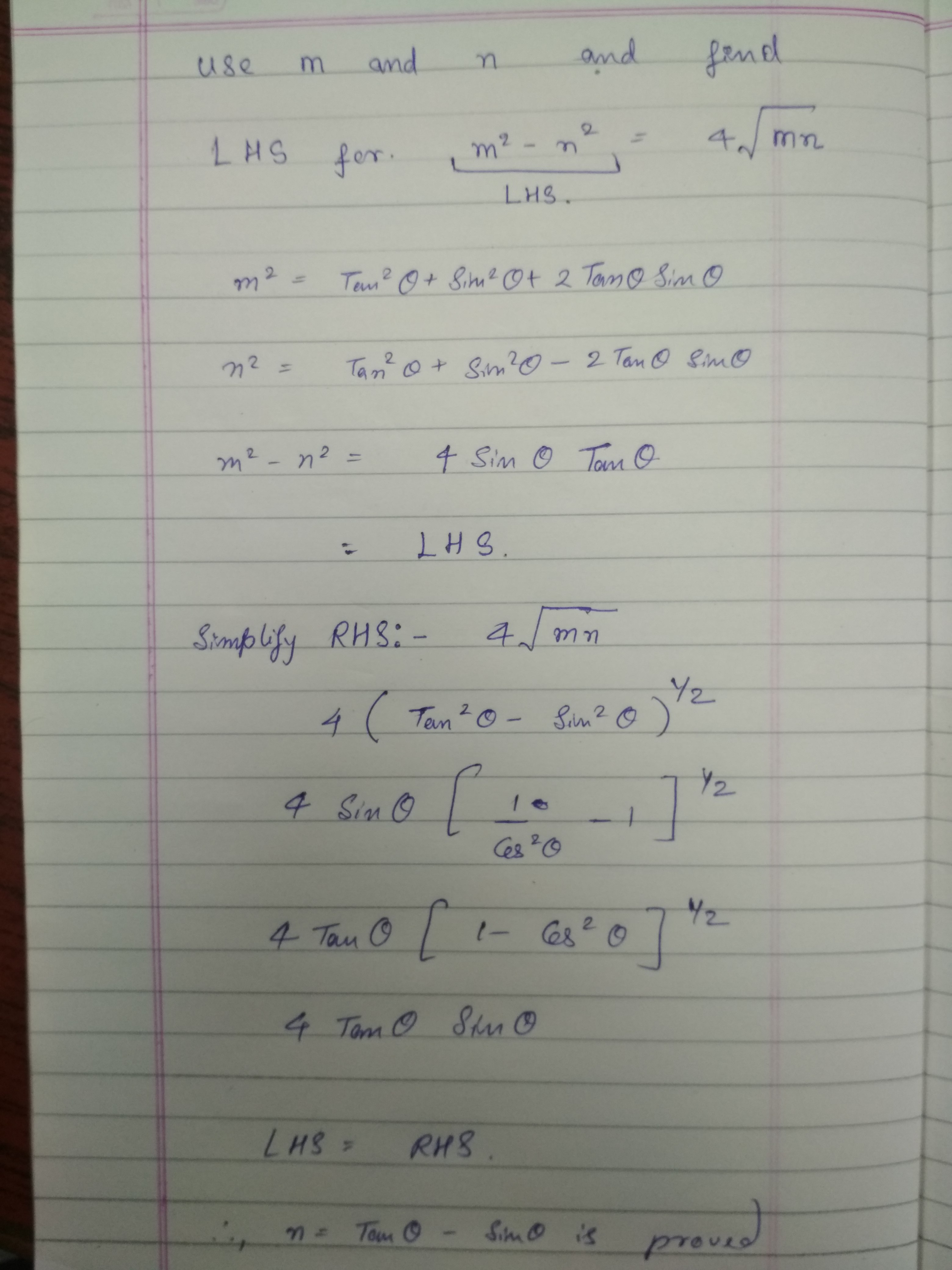×#### Thank you for registering.

One of our academic counsellors will contact you within 1 working day.

Click to Chat

1800-1023-196

+91-120-4616500

CART 0

• 0

MY CART (5)

Use Coupon: CART20 and get 20% off on all online Study Material

ITEM
DETAILS
MRP
DISCOUNT
FINAL PRICE
Total Price: Rs.

There are no items in this cart.
Continue Shopping
```
If m=tanA+sinA and m 2 -n 2 =4√mn Then show that, n=tanA-sinA

```
one year ago

```							let's consider the expression:  (tanA + sinA)² - (tanA - sinA)² =  let's factor it as a difference of squares:  [(tanA + sinA) + (tanA - sinA)][(tanA + sinA) - (tanA - sinA)] =  (tanA + sinA + tanA - sinA)(tanA + sinA - tanA - sinA) =  (2tanA)(2sinA) =  4tanA sinA =  (writing tanA as sinA /cosA)  4(sinA/cosA) sinA =  4(sin²A /cosA)  we can rewrite this (on condition that cosA is positive) as:  4√(sin²A /cosA)² =  4√[(sin²A)² /cos²A] =  let's rewrite the numerator of the radicand as:  4√{[(sin²A)(sin²A)] /cos²A} =  let's replace the second factor in the numerator with 1 - cos²A:  4√{[sin²A (1 - cos²A)] /cos²A} =  4√[(sin²A /cos²A)(1 - cos²A)]} =  (expanding and simplifying)  4√[(sin²A /cos²A) - (sin²A /cos²A) cos²A] =  4√[(sinA /cosA)² - sin²A] =  4√(tan²A - sin²A) =  (factoring the radicand as a difference of squares)  4√[(tanA + sinA)(tanA - sinA)]  summing up, we have:  (tanA + sinA)² - (tanA - sinA)² = 4√[(tanA + sinA)(tanA - sinA)]  where tanA + sinA = m:  m² - (tanA - sinA)² = 4√[m (tanA - sinA)]  on the other hand, we know that:  m² - n² = 4√(m n)  then, comparing the two expressions, we conclude that:  tanA - sinA = n
```
one year agoSaurabh Koranglekar
10233 Points
``````
one year ago
Think You Can Provide A Better Answer ?

## Other Related Questions on Trigonometry

View all Questions »### Course Features

• 731 Video Lectures
• Revision Notes
• Previous Year Papers
• Mind Map
• Study Planner
• NCERT Solutions
• Discussion Forum
• Test paper with Video Solution### Course Features

• 31 Video Lectures
• Revision Notes
• Test paper with Video Solution
• Mind Map
• Study Planner
• NCERT Solutions
• Discussion Forum
• Previous Year Exam Questions